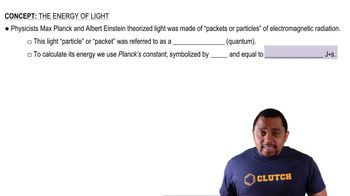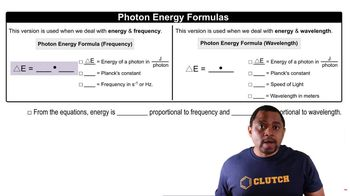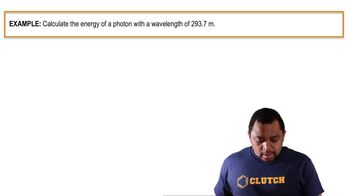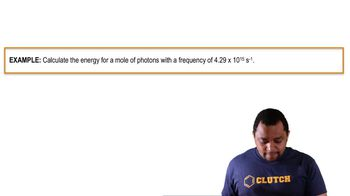Start typing, then use the up and down arrows to select an option from the list.
12:59 minutes
Problem 58
Textbook Question

# According to the equation for the Balmer line spectrum of hydrogen, a value of n = 3 gives a red spectral line at 656.3 nm, a value of n = 4 gives a green line at 486.1 nm, and a value of n = 5 gives a blue line at 434.0 nm. Calculate the energy in kilojoules per mole of the radiation corresponding to each of these spectral lines.Verified Solution
This video solution was recommended by our tutors as helpful for the problem above.
177views

### Watch next

Master The Energy of Light with a bite sized video explanation from Jules Bruno

Start learning00:5001:4001:0800:4101:35